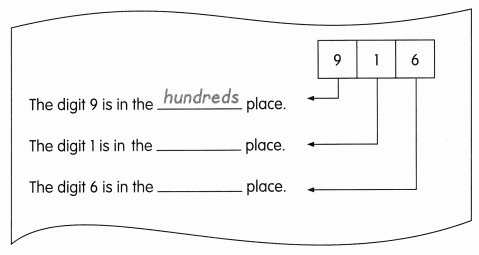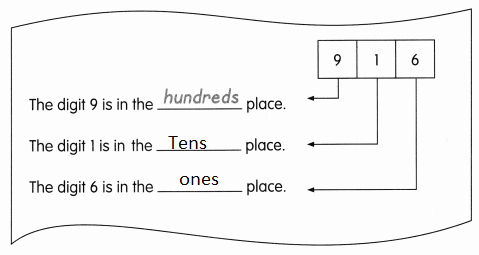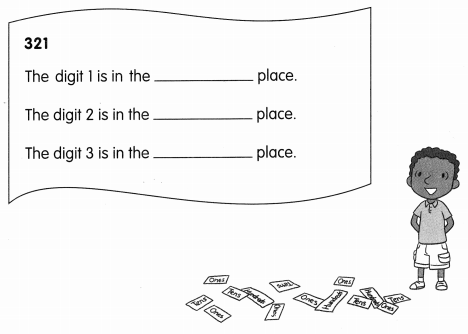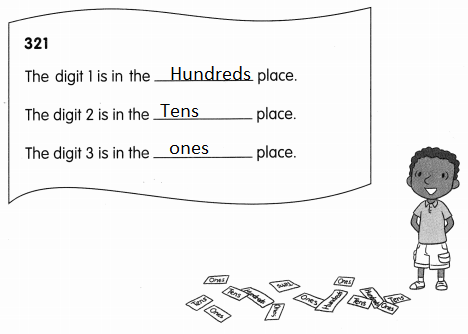# Math in Focus Grade 2 Chapter 1 Practice 2 Answer Key Place Value

Go through the Math in Focus Grade 2 Workbook Answer Key Chapter 1 Practice 2 Place Value to finish your assignments.

## Math in Focus Grade 2 Chapter 1 Practice 2 Answer Key Place Value

Look at the place-value charts.
Then write the numbers in standard form, word form, and expanded form.

Example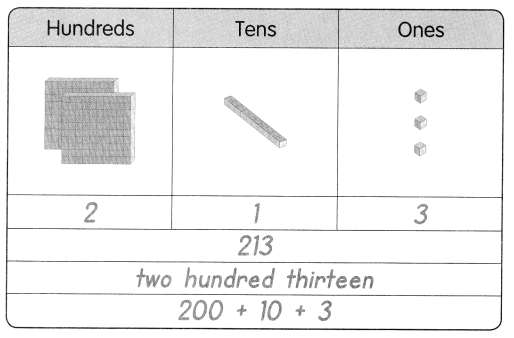Question 1.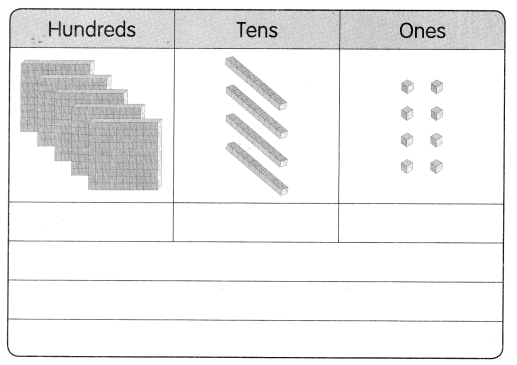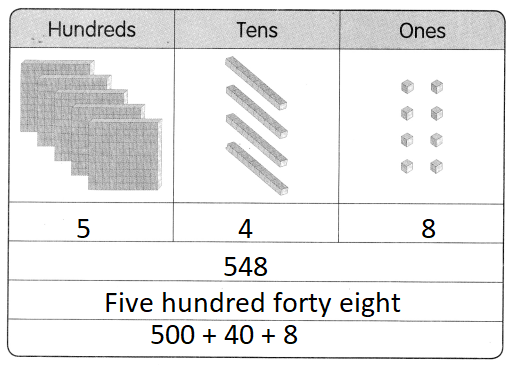Explanation:
1 cube = 100
1 line = 10
1 dot = 1
5 x 100 = 500
4 x 10 = 40
8 x 1 = 8
500 + 40 + 8 = 548

Write the numbers in standard form, word form, and expanded form.

Question 2.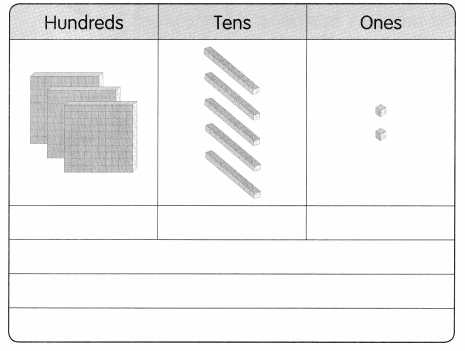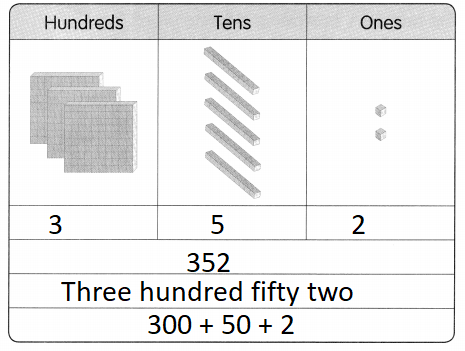Explanation:
1 cube = 100
1 line = 10
1 dot = 1
3 x 100 = 300
5 x 10 = 50
1 x 2 = 2
300 + 50 + 2 = 352

Question 3.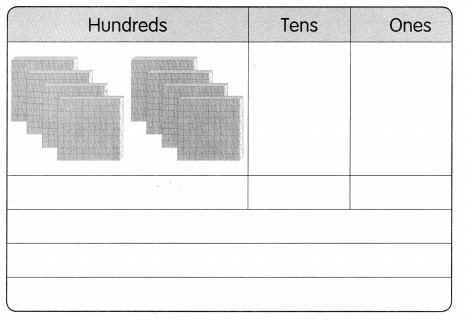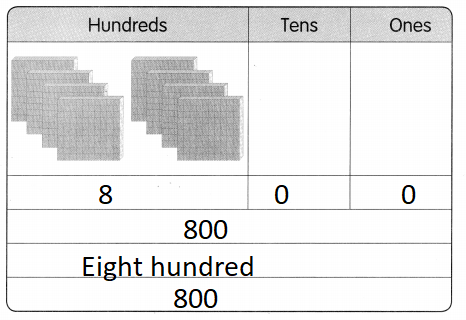Explanation:
1 cube = 100
1 line = 10
1 dot = 1
8 x 100 = 800

Write the numbers in standard form, word form, and expanded form.

Question 4.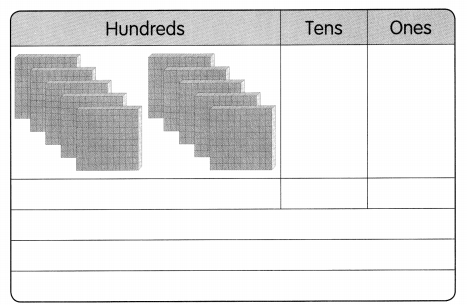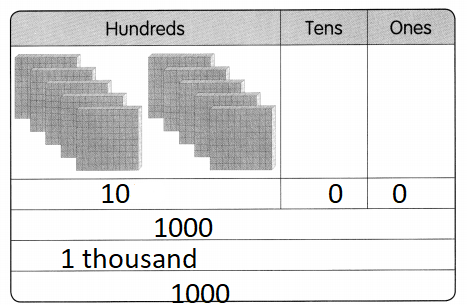Explanation:
1 cube = 100
1 line = 10
1 dot = 1
10 x 100 = 1000

Write the missing numbers and words.

Example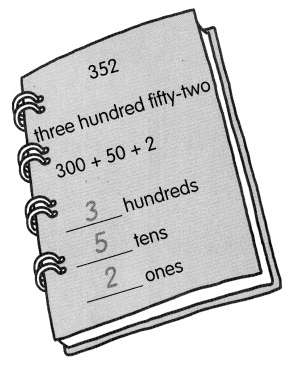Write the missing numbers and words.

Question 5.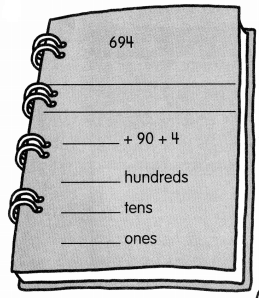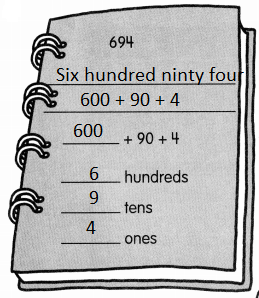Explanation:
Given number is in standard form
written in expanded form and word form

Question 6.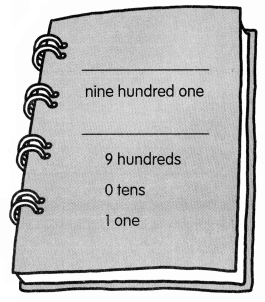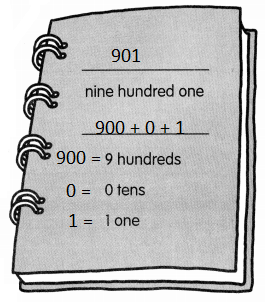Explanation:
Given number is in standard form
written in expanded form and word form

Question 7.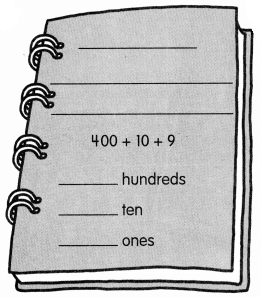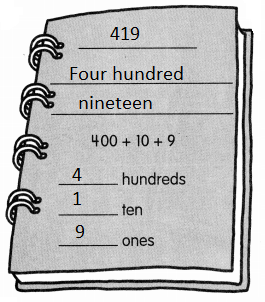Explanation:
Given number is in standard form
written in expanded form and word form

Question 8.
Color these bubbles. Use the same color for bubbles that show the same number.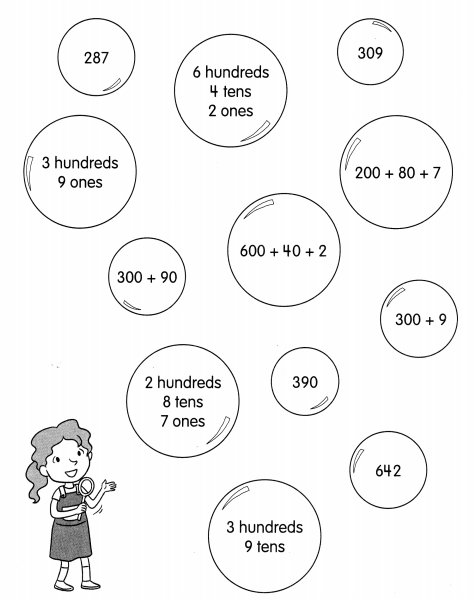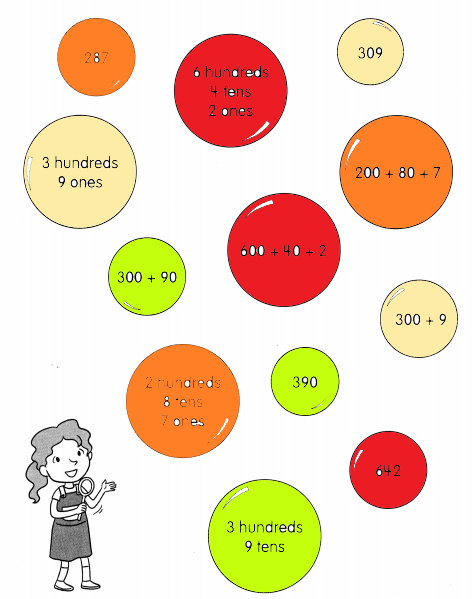Explanation:
standard form is matched with the expanded form

Write hundreds, tens, or ones.

Question 9.